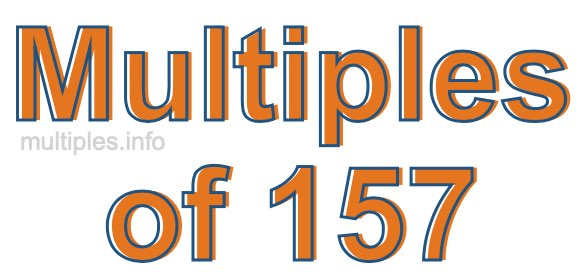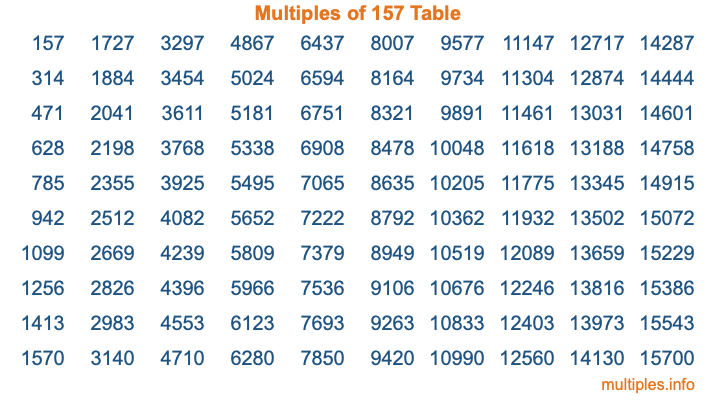Multiples of 157Welcome to the Multiples of 157 page. Here we will first teach you everything you will ever need to know about the multiples of 157, and then give you a study guide summary of everything we taught you to make sure you remember it all. Use this page to look up facts and learn information about the multiples of 157. This page will make you a multiples of one hundred fifty-seven expert!

Definition of Multiples of 157
Multiples of 157 are all the numbers that when divided by 157 equal an integer. Each of the multiples of 157 are called a multiple. A multiple of 157 is created by multiplying 157 by an integer.

Therefore, to create a list of multiples of 157, you start with 1 multiplied by 157, then 2 multiplied by 157, then 3 multiplied by 157, and so on for as long as you want. Thus, the list of the first five multiples of 157 is 157, 314, 471, 628, and 785. To see a larger list of multiples of 157, see the printable image of Multiples of 157 further down on this page. We also have a category where you can choose any nth multiple of 157.

Multiples of 157 Checker
The Multiples of 157 Checker below checks to see if any number of your choice is a multiple of 157. In other words, it checks to see if there is any number (integer) that when multiplied by 157 will equal your number. To do that, we divide your number by 157. If the the quotient is an integer, then your number is a multiple of 157.

Is  a multiple of 157?

Least Common Multiple of 157 and ...
A Least Common Multiple (LCM) is the lowest multiple that two or more numbers have in common. This is also called the smallest common multiple or lowest common multiple and is useful to know when you are adding our subtracting fractions. Enter one or more numbers below (157 is already entered) to find the LCM.

Check out our LCM Calculator if you need more details about the Least Common Multiple or if you need the LCM for different numbers for adding and subtraction fractions.

nth Multiple of 157
As we stated above, 157 is the first multiple of 157, 314 is the second multiple of 157, 471 is the third multiple of 157, and so on. Enter a number below to find the nth multiple of 157.

th multiple of 157

Multiples of 157 vs Factors of 157
157 is a multiple of 157 and a factor of 157, but that is where the similarities end. All postive multiples of 157 are 157 or greater than 157. All positive factors of 157 are 157 or less than 157.

Below is the beginning list of multiples of 157 and the factors of 157 so you can compare:

Multiples of 157: 157, 314, 471, 628, 785, etc.

Factors of 157: 1, 157

As you can see, the multiples of 157 are all the numbers that you can divide by 157 to get a whole number. The factors of 157, on the other hand, are all the whole numbers that you can multiply by another whole number to get 157.

It's also interesting to note that if a number (x) is a factor of 157, then 157 will also be a multiple of that number (x).

Multiples of 157 vs Divisors of 157
The divisors of 157 are all the integers that 157 can be divided by evenly. Below is a list of the divisors of 157.

Divisors of 157: 1, 157

The interesting thing to note here is that if you take any multiple of 157 and divide it by a divisor of 157, you will see that the quotient is an integer.

Multiples of 157 Table
Below is an image of the first 100 multiples of 157 in a table. The table is in chronological order, column by column. The first column has the first ten multiples of 157, the second column has the next ten multiples of 157, and so on.The Multiples of 157 Table is also referred to as the 157 Times Table or Times Table of 157. You are welcome to print out our table for your studies.

Negative Multiples of 157
Although not often discussed or needed in math, it is worth mentioning that you can make a list of negative multiples of 157 by multiplying 157 by -1, then by -2, then by -3, and so on, to get the following list of negative multiples of 157:

-157, -314, -471, -628, -785, etc.

Multiples of 157 Summary
Below is a summary of important Multiples of 157 facts that we have discussed on this page. To retain the knowledge on this page, we recommend that you read through the summary and explain to yourself or a study partner why they hold true.

There are an infinite number of multiples of 157.

A multiple of 157 divided by 157 will equal a whole number.

157 divided by a factor of 157 equals a divisor of 157.

The nth multiple of 157 is n times 157.

The largest factor of 157 is equal to the first positive multiple of 157.

157 is a multiple of every factor of 157.

157 is a multiple of 157.

A multiple of 157 divided by a divisor of 157 equals an integer.

157 divided by a divisor of 157 equals a factor of 157.

Any integer times 157 will equal a multiple of 157.

Multiples of a Number
Here you can get the multiples of another number, all with the same attention to detail as we did for multiples of 157 on this page.

Multiples of
Multiples of 158
Did you find our page about multiples of one hundred fifty-seven educational? Do you want more knowledge? Check out the multiples of the next number on our list!